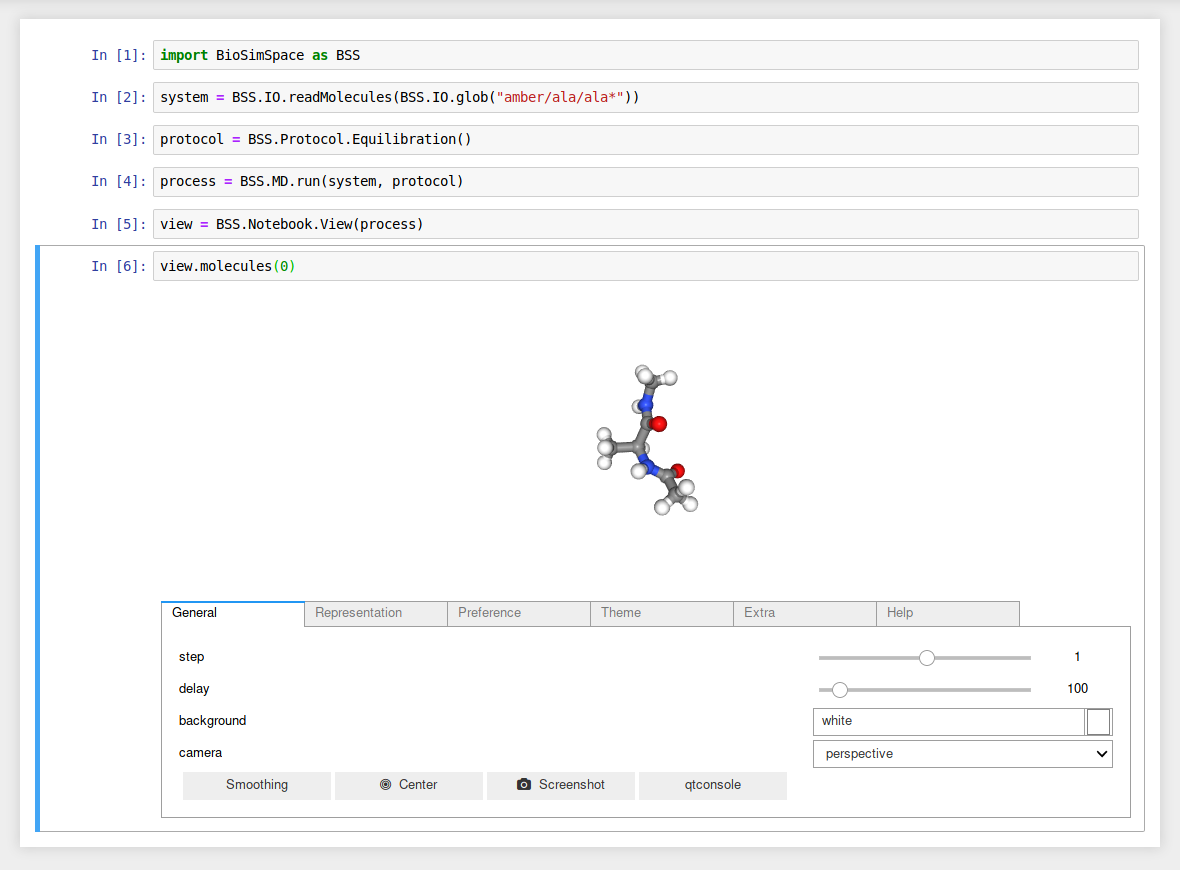# BioSimSpace.Notebook#

The Notebook package contains tools that are used when BioSimSpace is run interactively, e.g. within a Jupyter notebook.

## Functions#

 `plot`([x, y, xerr, yerr, xlabel, ylabel, ...]) A simple function to create x/y plots with matplotlib. `plotContour`(x, y, z[, xlabel, ylabel, zlabel]) A simple function to create two-dimensional contour plots with matplotlib. `plotOverlapMatrix`(overlap[, ...]) Plot the overlap matrix from a free-energy perturbation analysis.

Examples

Generate a line graph using two lists of data.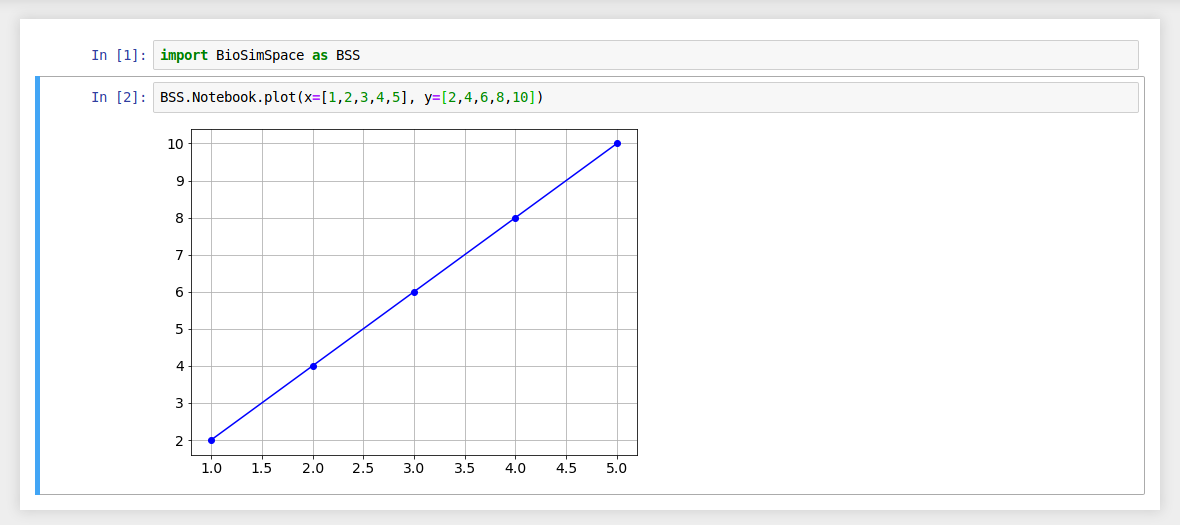If no argument is passed for the `x` data then each `y` data value is plotted against its list index.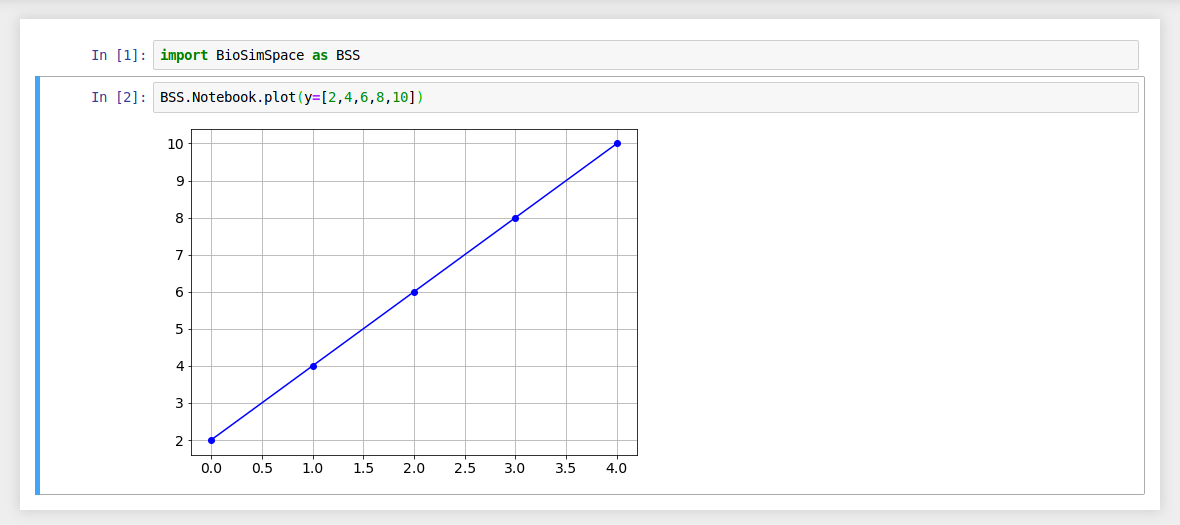Use the `xlabel` and `ylabel` arguments to add labels to your plots.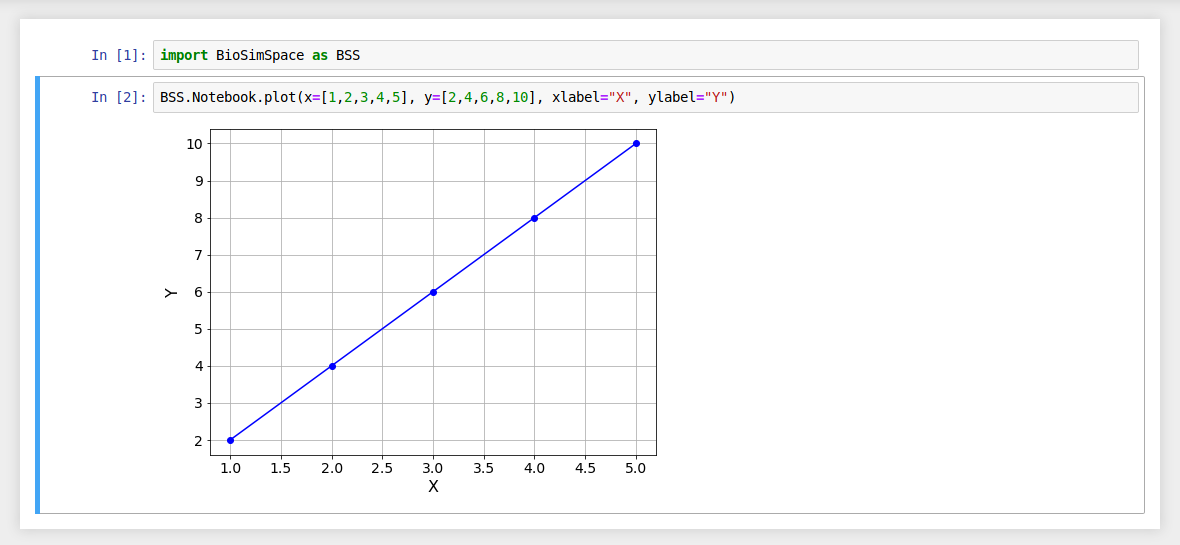Error bars can be added using `xerror` and `yerror`.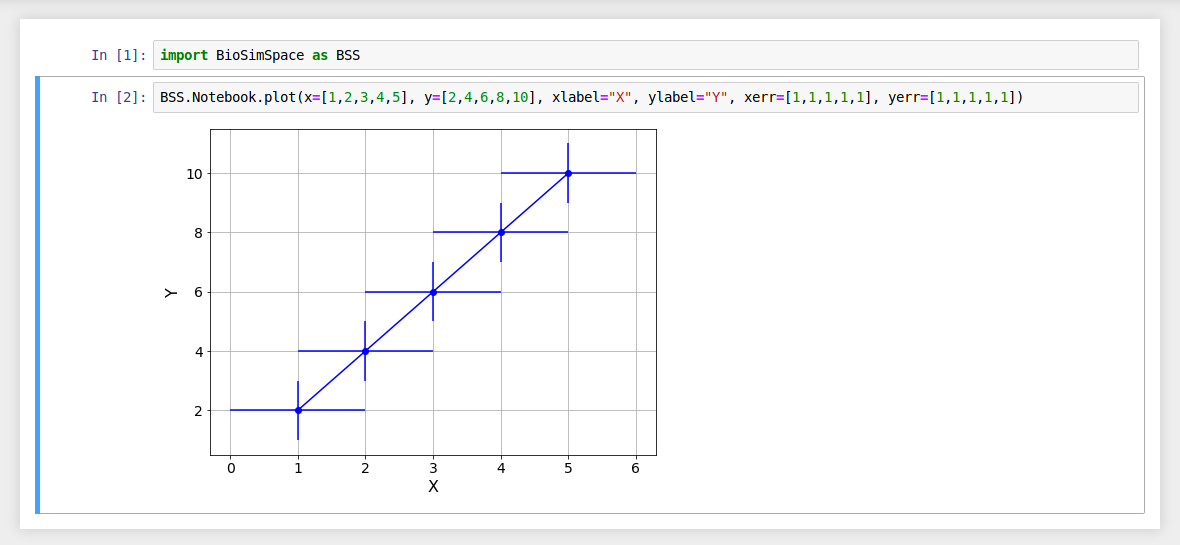It is possible to generate plots from the output of a real-time simulation. Where functions return time-series data containing BioSimSpace.Types, then axis labels will be automatically generated. (The `xlabel` and `ylabel` still take precedence.)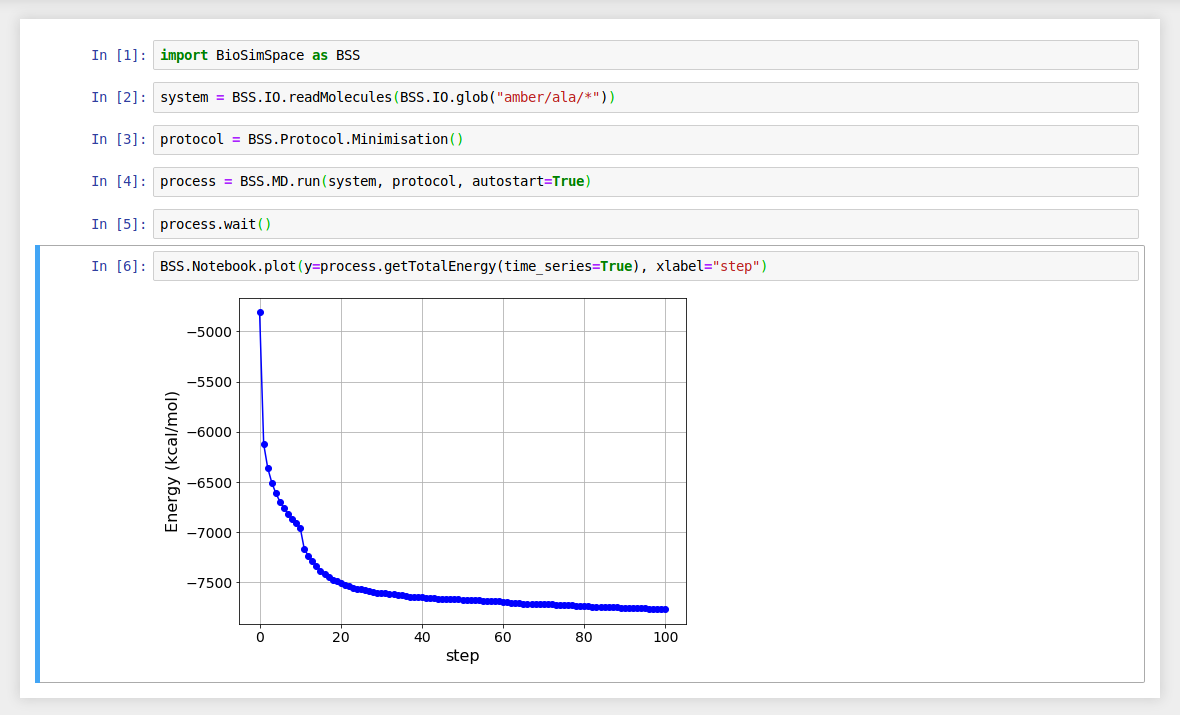## Classes#

 `View`(handle[, property_map, is_lambda1]) A class for handling interactive molecular visualisations.

Examples

Load and visualise a molecular system.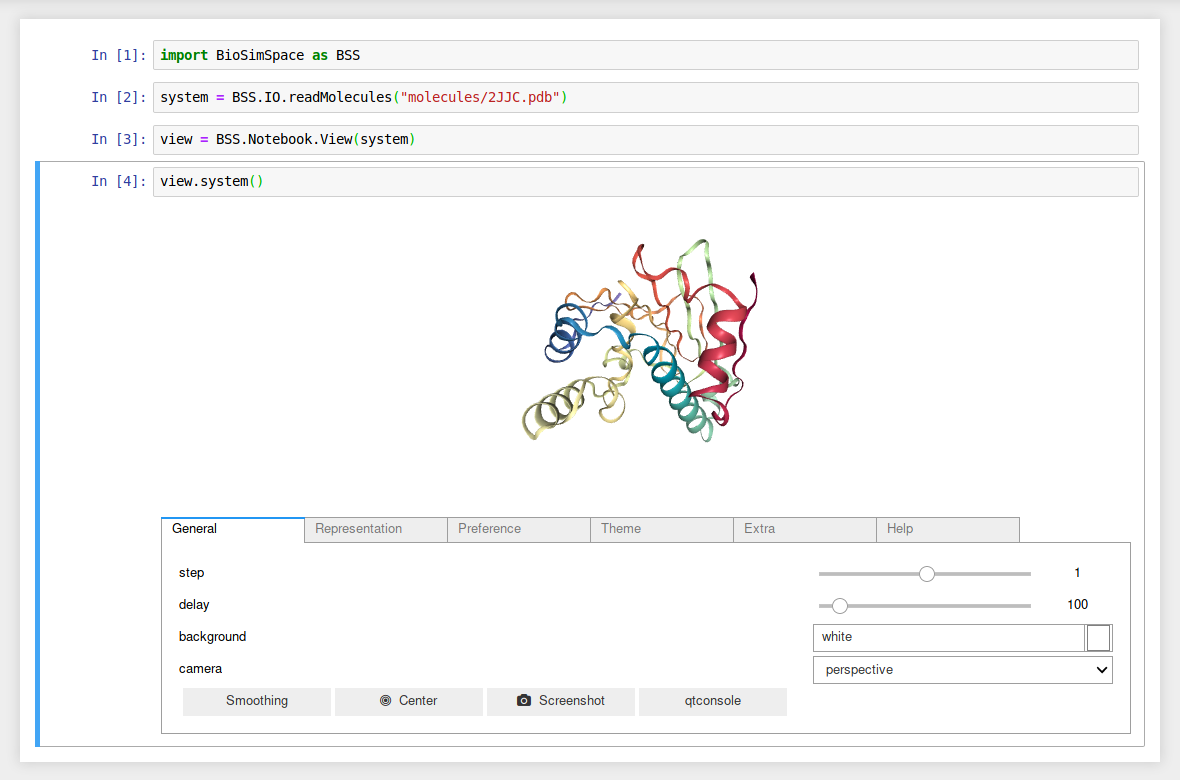Attach a `View` to a running molecular dynamics `Process` and visualise the first molecule in the system from the latest configuration in real-time.